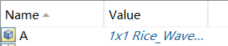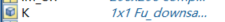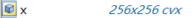How to use an operator of matrix in CVX!

Hello, I am facing some errors when used CVX to optimize a convex problem below

where K and A is an operator of matrix that only support the formal data type in MATLAB, such as double, single, int8, uint8, int16, uint16, int32, uint32, and logical. However, The data type of variable x is cvx. The type of K, A and x in the workspace of MATLAB is shown asThe error I am facing is that

How can I use such operators in CVX? Any suggestion can be helpful.

I don’t know whether you can All CVX code needs to follow its rules.

If the problem is really a convex optimization problem (this forum is full of false claims of convexity by posters), then perhaps it can be entered into CVX, but not using the unsupported functions you call, which apparently produce unsupported object types. If K and A wind up being known input matrices having double precision elements, or you can convert them to that form, then they can be used in CVX. If K or A is some nonlinear function of the variable x, then your problem is (likely) non-convex.